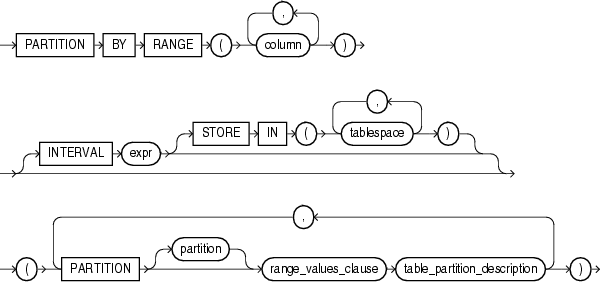# Oracle Partition - Partitioning key(s)

The partitioning key(s) is an ordered list of columns.

## Restrictions

The list columns must contain only columns (no expression). If you want to use a function in the partition key, you need to create a virtual_column.

## Example

### Virtual Column

• The partition key chosen is the D_TIME_CODE column with the char datatype holding a calendar prefix with a week code. Example of data FW201602.
• The scheme chosen is a Range Interval Partitioning.
• The data type of the table column must not change

In this configuration, it's not possible to create directly a Range Interval Partitioning because the key column must be a number or a date. Therefore we create a virtual column transforming the character to a number.

``````create table EXAMPLE (
D_TIME_CODE        VARCHAR2(10 CHAR),
D_TIME_CODE_V    NUMBER GENERATED ALWAYS AS (  substr(D_TIME_CODE,3) ) VIRTUAL
)
PARTITION BY RANGE (D_TIME_CODE_V)
INTERVAL(1)
(
PARTITION p1 VALUES LESS THAN ( 201014 )
) ```
```

Discover MoreOracle Partition - Range Interval Partitioning

A range partitioning where the database automatically creates partitions for a specified . At least one range partition using the PARTITION clause. The partitioning key can only be asingle column...Oracle Partition - Range partitioning (less than)

The table is partitioned by ranges of values from one (or more) columns. See Reference Range Partition where:...